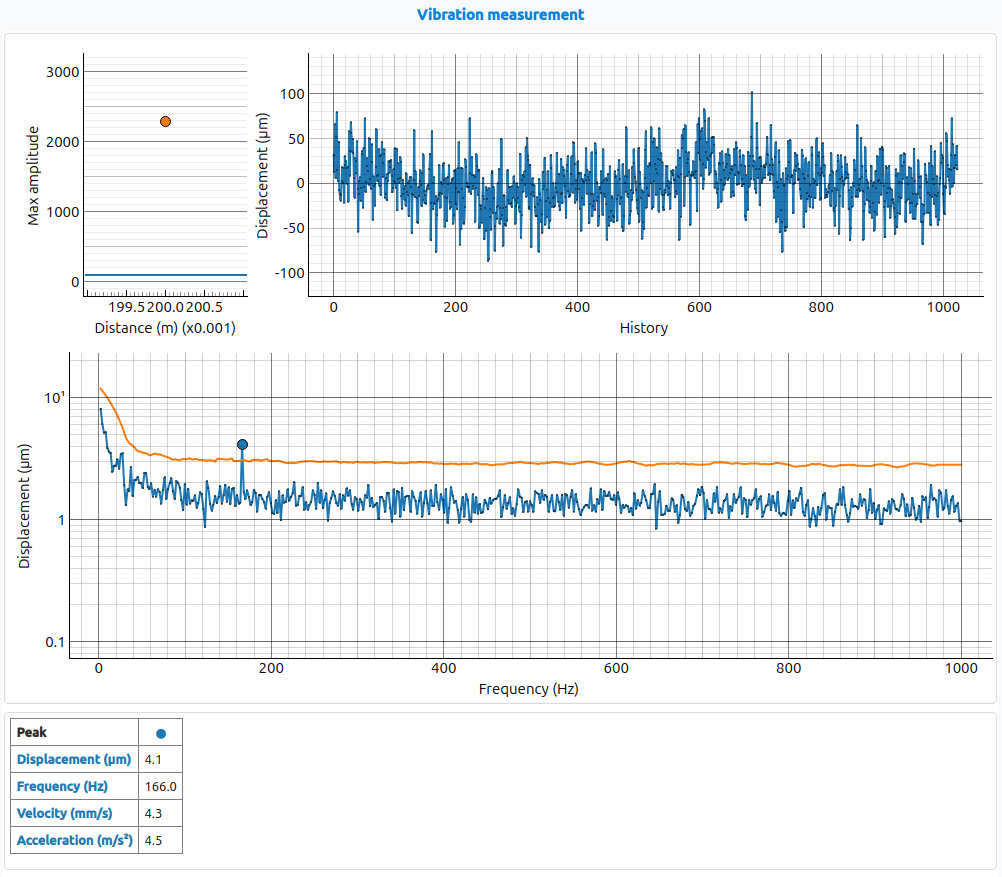# Vibration measurement#

This vibration measurement application illustrates how the a121 sensor can be used to estimate the frequency content of a vibrating object, measured at a distance.

The Sparse IQ service produces complex data samples where the amplitude reflects the amount of returning energy and the phase the relative timing between the transmitted and received pulse. The phase can be used to detect small displacements. The vibration measurement application takes advantage of this, by forming a time series of the measured phase in a single distance point, which is then analyzed using an FFT. See Interpreting radar data for more information about the produced data.

The length of the time series is determine by the processor configuration parameter `time_series_length`. A longer time series will give more points in the resulting spectrum. The frequency resolution is given by the ratio of the sweep rate and the number of points in the time series.

The frequency resolution of the spectrum is determine by the sweep rate(the time between consecutive sweeps). The sweep rate can be changed through the GUI, or be specified in the sensor configuration if running the application from a script.

The processor configuration also has a time constant, `lp_coeff`, applied together with an exponential filter, providing the possibility to time filter the calculated spectrum.

The GUI, shown below, has three plots. The upper left indicates the amplitude at the measured point. The purpose of this is to provide visual feedback on whether or not the object is located at the right distance from the sensor. With no object in front of the sensor, the value should be close to zero. When moving an object into the correct location, the value increases. Next, the upper right plot shows the displacement of the measured object. Here the phase has been converted to mm, indicating the physical displacement of the object. Lastly, the lower plot shows the calculated spectrum. In this case, the vibrating object has a dominant frequency at 440 Hz with a possible harmonic at 880 Hz.## Configuration parameters#

class acconeer.exptool.a121.algo.vibration._processor.ProcessorConfig(*, time_series_length: int = 1024, lp_coeff: float = 0.95, sensitivity: float = 10.0, amplitude_threshold: float = 100.0)#
time_series_length: int#

Length of time series.

lp_coeff: float#

Specify filter coefficient of exponential filter.

sensitivity: float#

Specify threshold sensitivity.

amplitude_threshold: float#

Specify minimum amplitude for calculating vibration.